# 5.3 Graphs of polynomial functions  (Page 9/13)

 Page 9 / 13

$f\left(x\right)={x}^{5}-2x,\text{\hspace{0.17em}}$ between $\text{\hspace{0.17em}}x=1\text{\hspace{0.17em}}$ and $\text{\hspace{0.17em}}x=2.$

$f\left(x\right)=-{x}^{4}+4,\text{\hspace{0.17em}}$ between $\text{\hspace{0.17em}}x=1\text{\hspace{0.17em}}$ and $\text{\hspace{0.17em}}x=3$ .

$f\left(1\right)=3\text{\hspace{0.17em}}$ and $\text{\hspace{0.17em}}f\left(3\right)=–77.\text{\hspace{0.17em}}$ Sign change confirms.

$f\left(x\right)=-2{x}^{3}-x,\text{\hspace{0.17em}}$ between $\text{\hspace{0.17em}}x=–1\text{\hspace{0.17em}}$ and $\text{\hspace{0.17em}}x=1.$

$f\left(x\right)={x}^{3}-100x+2,\text{\hspace{0.17em}}$ between $\text{\hspace{0.17em}}x=0.01\text{\hspace{0.17em}}$ and $\text{\hspace{0.17em}}x=0.1$

$f\left(0.01\right)=1.000001\text{\hspace{0.17em}}$ and $\text{\hspace{0.17em}}f\left(0.1\right)=–7.999.\text{\hspace{0.17em}}$ Sign change confirms.

For the following exercises, find the zeros and give the multiplicity of each.

$f\left(x\right)={\left(x+2\right)}^{3}{\left(x-3\right)}^{2}$

$f\left(x\right)={x}^{2}{\left(2x+3\right)}^{5}{\left(x-4\right)}^{2}$

0 with multiplicity 2, $\text{\hspace{0.17em}}-\frac{3}{2}\text{\hspace{0.17em}}$ with multiplicity 5, 4 with multiplicity 2

$f\left(x\right)={x}^{3}{\left(x-1\right)}^{3}\left(x+2\right)$

$f\left(x\right)={x}^{2}\left({x}^{2}+4x+4\right)$

0 with multiplicity 2, –2 with multiplicity 2

$f\left(x\right)={\left(2x+1\right)}^{3}\left(9{x}^{2}-6x+1\right)$

$f\left(x\right)={\left(3x+2\right)}^{5}\left({x}^{2}-10x+25\right)$

$-\frac{2}{3}\text{\hspace{0.17em}}\text{with}\text{\hspace{0.17em}}\text{multiplicity}\text{\hspace{0.17em}}5\text{,}\text{\hspace{0.17em}}5\text{\hspace{0.17em}}\text{with}\text{\hspace{0.17em}}\text{multiplicity}\text{\hspace{0.17em}}\text{2}$

$f\left(x\right)=x\left(4{x}^{2}-12x+9\right)\left({x}^{2}+8x+16\right)$

$f\left(x\right)={x}^{6}-{x}^{5}-2{x}^{4}$

$\text{0}\text{\hspace{0.17em}}\text{with}\text{\hspace{0.17em}}\text{multiplicity}\text{\hspace{0.17em}}4\text{,}\text{\hspace{0.17em}}2\text{\hspace{0.17em}}\text{with}\text{\hspace{0.17em}}\text{multiplicity}\text{\hspace{0.17em}}1\text{,}\text{\hspace{0.17em}}–\text{1}\text{\hspace{0.17em}}\text{with}\text{\hspace{0.17em}}\text{multiplicity}\text{\hspace{0.17em}}1$

$f\left(x\right)=3{x}^{4}+6{x}^{3}+3{x}^{2}$

$f\left(x\right)=4{x}^{5}-12{x}^{4}+9{x}^{3}$

$\frac{3}{2}\text{\hspace{0.17em}}$ with multiplicity 2, 0 with multiplicity 3

$f\left(x\right)=2{x}^{4}\left({x}^{3}-4{x}^{2}+4x\right)$

$f\left(x\right)=4{x}^{4}\left(9{x}^{4}-12{x}^{3}+4{x}^{2}\right)$

$\text{0}\text{\hspace{0.17em}}\text{with}\text{\hspace{0.17em}}\text{multiplicity}\text{\hspace{0.17em}}6\text{,}\text{\hspace{0.17em}}\frac{2}{3}\text{\hspace{0.17em}}\text{with}\text{\hspace{0.17em}}\text{multiplicity}\text{\hspace{0.17em}}2$

## Graphical

For the following exercises, graph the polynomial functions. Note $\text{\hspace{0.17em}}x\text{-}$ and $\text{\hspace{0.17em}}y\text{-}$ intercepts, multiplicity, and end behavior.

$f\left(x\right)={\left(x+3\right)}^{2}\left(x-2\right)$

$g\left(x\right)=\left(x+4\right){\left(x-1\right)}^{2}$

x -intercepts, $\left(1, 0\right)$ with multiplicity 2, with multiplicity 1, $y\text{-}$ intercept As $\phantom{\rule{0.2em}{0ex}}x\to -\infty ,\phantom{\rule{0.2em}{0ex}}f\left(x\right)\to -\infty ,\text{\hspace{0.17em}}\text{as}\phantom{\rule{0.2em}{0ex}}x\to \infty ,\phantom{\rule{0.2em}{0ex}}f\left(x\right)\to \infty .$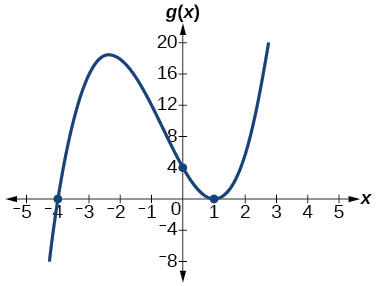$h\left(x\right)={\left(x-1\right)}^{3}{\left(x+3\right)}^{2}$

$k\left(x\right)={\left(x-3\right)}^{3}{\left(x-2\right)}^{2}$

x -intercepts $\text{\hspace{0.17em}}\left(3,0\right)\text{\hspace{0.17em}}$ with multiplicity 3, $\text{\hspace{0.17em}}\left(2,0\right)\text{\hspace{0.17em}}$ with multiplicity 2, $\text{\hspace{0.17em}}y\text{-}$ intercept $\text{\hspace{0.17em}}\left(0,–108\right).\text{\hspace{0.17em}}$ As $x\to -\infty ,\phantom{\rule{0.2em}{0ex}}f\left(x\right)\to -\infty ,\phantom{\rule{0.2em}{0ex}}\text{as}\phantom{\rule{0.2em}{0ex}}x\to \infty ,\phantom{\rule{0.2em}{0ex}}f\left(x\right)\to \infty .$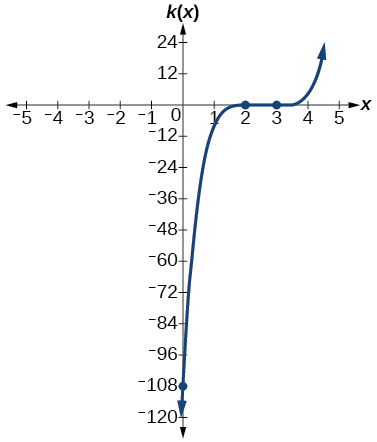$m\left(x\right)=-2x\left(x-1\right)\left(x+3\right)$

$n\left(x\right)=-3x\left(x+2\right)\left(x-4\right)$

x -intercepts with multiplicity 1, $\text{\hspace{0.17em}}y\text{-}$ intercept As $x\to -\infty ,\phantom{\rule{0.2em}{0ex}}f\left(x\right)\to \infty ,\phantom{\rule{0.2em}{0ex}}\text{as}\phantom{\rule{0.2em}{0ex}}x\to \infty ,\phantom{\rule{0.2em}{0ex}}f\left(x\right)\to -\infty .$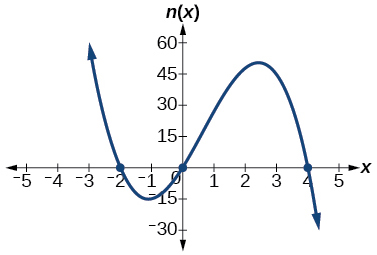For the following exercises, use the graphs to write the formula for a polynomial function of least degree.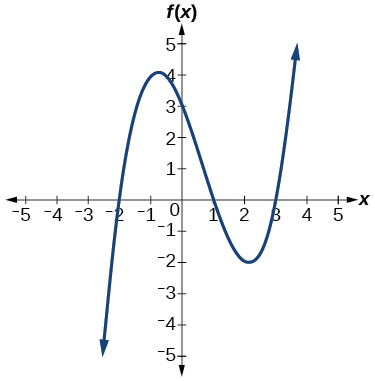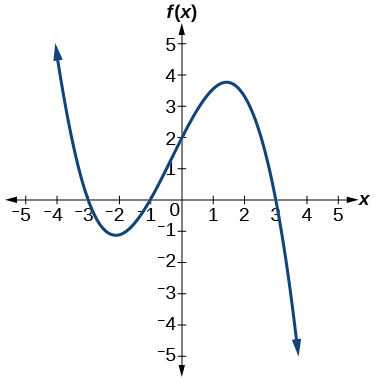$f\left(x\right)=-\frac{2}{9}\left(x-3\right)\left(x+1\right)\left(x+3\right)$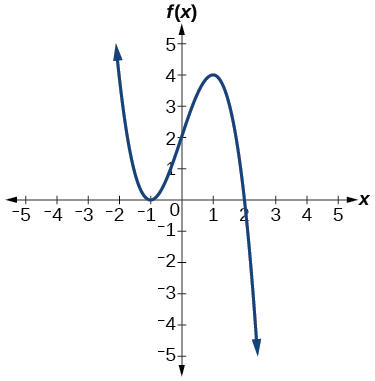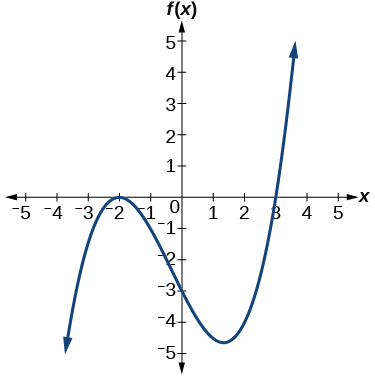$f\left(x\right)=\frac{1}{4}{\left(x+2\right)}^{2}\left(x-3\right)$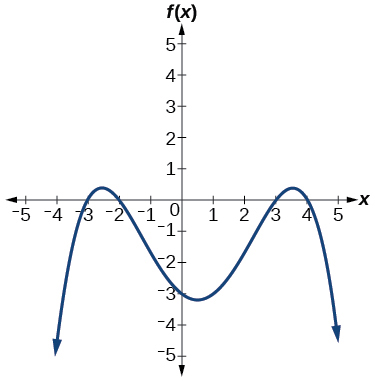For the following exercises, use the graph to identify zeros and multiplicity.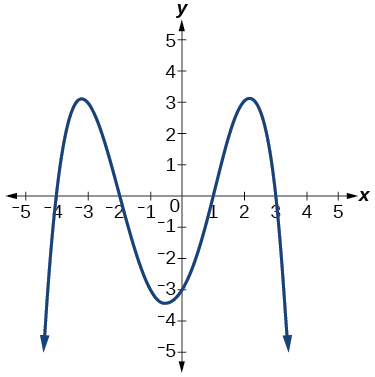–4, –2, 1, 3 with multiplicity 1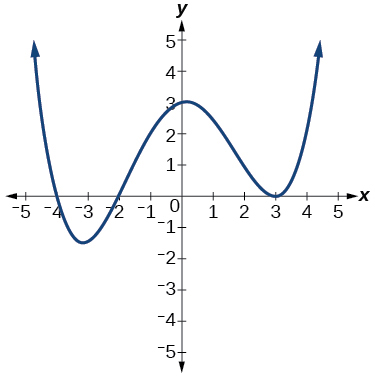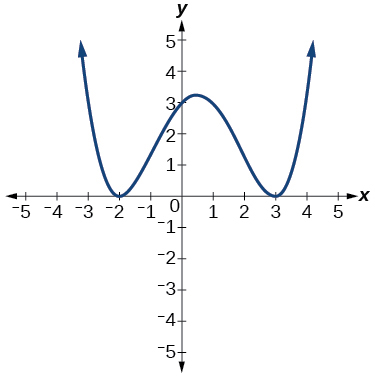–2, 3 each with multiplicity 2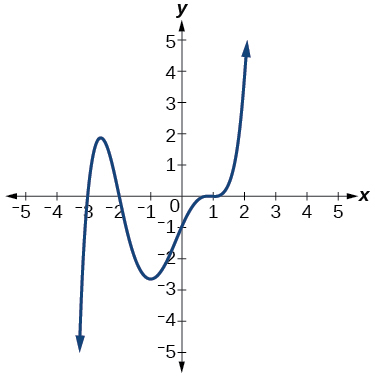For the following exercises, use the given information about the polynomial graph to write the equation.

Degree 3. Zeros at $\text{\hspace{0.17em}}x=–2,$ $\text{\hspace{0.17em}}x=1,\text{\hspace{0.17em}}$ and $\text{\hspace{0.17em}}x=3.\text{\hspace{0.17em}}$ y -intercept at $\text{\hspace{0.17em}}\left(0,–4\right).$

$f\left(x\right)=-\frac{2}{3}\left(x+2\right)\left(x-1\right)\left(x-3\right)$

Degree 3. Zeros at $\text{\hspace{0.17em}}x=\text{–5,}$ $\text{\hspace{0.17em}}x=–2,$ and $\text{\hspace{0.17em}}x=1.\text{\hspace{0.17em}}$ y -intercept at $\text{\hspace{0.17em}}\left(0,6\right)$

Degree 5. Roots of multiplicity 2 at $\text{\hspace{0.17em}}x=3\text{\hspace{0.17em}}$ and $\text{\hspace{0.17em}}x=1\text{\hspace{0.17em}}$ , and a root of multiplicity 1 at $\text{\hspace{0.17em}}x=–3.\text{\hspace{0.17em}}$ y -intercept at $\text{\hspace{0.17em}}\left(0,9\right)$

$f\left(x\right)=\frac{1}{3}{\left(x-3\right)}^{2}{\left(x-1\right)}^{2}\left(x+3\right)$

Degree 4. Root of multiplicity 2 at $\text{\hspace{0.17em}}x=4,\text{\hspace{0.17em}}$ and a roots of multiplicity 1 at $\text{\hspace{0.17em}}x=1\text{\hspace{0.17em}}$ and $\text{\hspace{0.17em}}x=–2.\text{\hspace{0.17em}}$ y -intercept at $\text{\hspace{0.17em}}\left(0,\text{–}3\right).$

Degree 5. Double zero at $\text{\hspace{0.17em}}x=1,\text{\hspace{0.17em}}$ and triple zero at $\text{\hspace{0.17em}}x=3.\text{\hspace{0.17em}}$ Passes through the point $\text{\hspace{0.17em}}\left(2,15\right).$

$f\left(x\right)=-15{\left(x-1\right)}^{2}{\left(x-3\right)}^{3}$

Degree 3. Zeros at $\text{\hspace{0.17em}}x=4,$ $\text{\hspace{0.17em}}x=3,$ and $\text{\hspace{0.17em}}x=2.\text{\hspace{0.17em}}$ y -intercept at $\text{\hspace{0.17em}}\left(0,-24\right).$

Degree 3. Zeros at $\text{\hspace{0.17em}}x=-3,$ $\text{\hspace{0.17em}}x=-2\text{\hspace{0.17em}}$ and $\text{\hspace{0.17em}}x=1.\text{\hspace{0.17em}}$ y -intercept at $\text{\hspace{0.17em}}\left(0,12\right).$

$f\left(x\right)=-2\left(x+3\right)\left(x+2\right)\left(x-1\right)$

Degree 5. Roots of multiplicity 2 at $\text{\hspace{0.17em}}x=-3\text{\hspace{0.17em}}$ and $\text{\hspace{0.17em}}x=2\text{\hspace{0.17em}}$ and a root of multiplicity 1 at $\text{\hspace{0.17em}}x=-2.$

y -intercept at

Degree 4. Roots of multiplicity 2 at $\text{\hspace{0.17em}}x=\frac{1}{2}\text{\hspace{0.17em}}$ and roots of multiplicity 1 at $\text{\hspace{0.17em}}x=6\text{\hspace{0.17em}}$ and $\text{\hspace{0.17em}}x=-2.$

y -intercept at $\text{\hspace{0.17em}}\left(0,18\right).$

$f\left(x\right)=-\frac{3}{2}{\left(2x-1\right)}^{2}\left(x-6\right)\left(x+2\right)$

Double zero at $\text{\hspace{0.17em}}x=-3\text{\hspace{0.17em}}$ and triple zero at $\text{\hspace{0.17em}}x=0.\text{\hspace{0.17em}}$ Passes through the point $\text{\hspace{0.17em}}\left(1,32\right).$

## Technology

For the following exercises, use a calculator to approximate local minima and maxima or the global minimum and maximum.

$f\left(x\right)={x}^{3}-x-1$

local max local min

$f\left(x\right)=2{x}^{3}-3x-1$

$f\left(x\right)={x}^{4}+x$

global min

$f\left(x\right)=-{x}^{4}+3x-2$

$f\left(x\right)={x}^{4}-{x}^{3}+1$

global min

## Extensions

For the following exercises, use the graphs to write a polynomial function of least degree.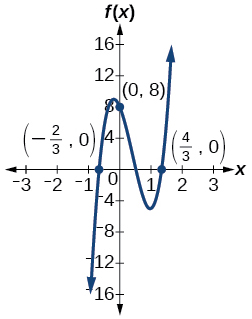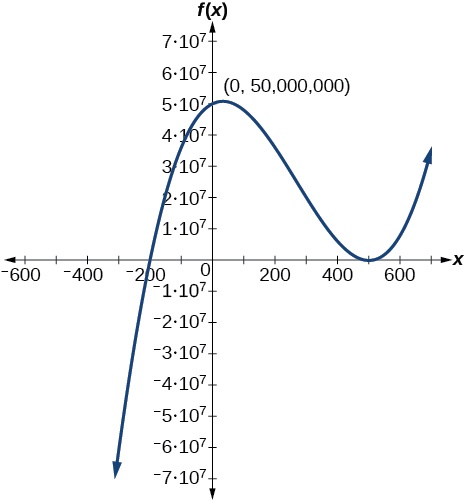$f\left(x\right)={\left(x-500\right)}^{2}\left(x+200\right)$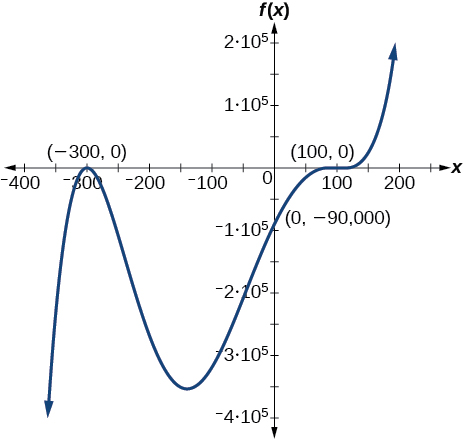## Real-world applications

For the following exercises, write the polynomial function that models the given situation.

A rectangle has a length of 10 units and a width of 8 units. Squares of $\text{\hspace{0.17em}}x\text{\hspace{0.17em}}$ by $\text{\hspace{0.17em}}x\text{\hspace{0.17em}}$ units are cut out of each corner, and then the sides are folded up to create an open box. Express the volume of the box as a polynomial function in terms of $\text{\hspace{0.17em}}x.$

$f\left(x\right)=4{x}^{3}-36{x}^{2}+80x$

Consider the same rectangle of the preceding problem. Squares of $\text{\hspace{0.17em}}2x\text{\hspace{0.17em}}$ by $\text{\hspace{0.17em}}2x\text{\hspace{0.17em}}$ units are cut out of each corner. Express the volume of the box as a polynomial in terms of $\text{\hspace{0.17em}}x.$

A square has sides of 12 units. Squares by units are cut out of each corner, and then the sides are folded up to create an open box. Express the volume of the box as a function in terms of $\text{\hspace{0.17em}}x.$

$f\left(x\right)=4{x}^{3}-36{x}^{2}+60x+100$

A cylinder has a radius of $\text{\hspace{0.17em}}x+2\text{\hspace{0.17em}}$ units and a height of 3 units greater. Express the volume of the cylinder as a polynomial function.

A right circular cone has a radius of $\text{\hspace{0.17em}}3x+6\text{\hspace{0.17em}}$ and a height 3 units less. Express the volume of the cone as a polynomial function. The volume of a cone is $\text{\hspace{0.17em}}V=\frac{1}{3}\pi {r}^{2}h\text{\hspace{0.17em}}$ for radius $\text{\hspace{0.17em}}r\text{\hspace{0.17em}}$ and height $\text{\hspace{0.17em}}h.$

$f\left(x\right)=\pi \left(9{x}^{3}+45{x}^{2}+72x+36\right)$

what are you up to?
nothing up todat yet
Miranda
hi
jai
hello
jai
Miranda Drice
jai
aap konsi country se ho
jai
which language is that
Miranda
I am living in india
jai
good
Miranda
what is the formula for calculating algebraic
I think the formula for calculating algebraic is the statement of the equality of two expression stimulate by a set of addition, multiplication, soustraction, division, raising to a power and extraction of Root. U believe by having those in the equation you will be in measure to calculate it
Miranda
state and prove Cayley hamilton therom
hello
Propessor
hi
Miranda
the Cayley hamilton Theorem state if A is a square matrix and if f(x) is its characterics polynomial then f(x)=0 in another ways evey square matrix is a root of its chatacteristics polynomial.
Miranda
hi
jai
hi Miranda
jai
thanks
Propessor
welcome
jai
What is algebra
algebra is a branch of the mathematics to calculate expressions follow.
Miranda
Miranda Drice would you mind teaching me mathematics? I think you are really good at math. I'm not good at it. In fact I hate it. 😅😅😅
Jeffrey
lolll who told you I'm good at it
Miranda
something seems to wispher me to my ear that u are good at it. lol
Jeffrey
lolllll if you say so
Miranda
but seriously, Im really bad at math. And I hate it. But you see, I downloaded this app two months ago hoping to master it.
Jeffrey
which grade are you in though
Miranda
oh woww I understand
Miranda
Jeffrey
Jeffrey
Miranda
how come you finished in college and you don't like math though
Miranda
gotta practice, holmie
Steve
if you never use it you won't be able to appreciate it
Steve
I don't know why. But Im trying to like it.
Jeffrey
yes steve. you're right
Jeffrey
so you better
Miranda
what is the solution of the given equation?
which equation
Miranda
I dont know. lol
Jeffrey
Miranda
Jeffrey
answer and questions in exercise 11.2 sums
how do u calculate inequality of irrational number?
Alaba
give me an example
Chris
and I will walk you through it
Chris
cos (-z)= cos z .
cos(- z)=cos z
Mustafa
what is a algebra
(x+x)3=?
6x
Obed
what is the identity of 1-cos²5x equal to?
__john __05
Kishu
Hi
Abdel
hi
Ye
hi
Nokwanda
C'est comment
Abdel
Hi
Amanda
hello
SORIE
Hiiii
Chinni
hello
Ranjay
hi
ANSHU
hiiii
Chinni
h r u friends
Chinni
yes
Hassan
so is their any Genius in mathematics here let chat guys and get to know each other's
SORIE
I speak French
Abdel
okay no problem since we gather here and get to know each other
SORIE
hi im stupid at math and just wanna join here
Yaona
lol nahhh none of us here are stupid it's just that we have Fast, Medium, and slow learner bro but we all going to work things out together
SORIE
it's 12
what is the function of sine with respect of cosine , graphically
tangent bruh
Steve
cosx.cos2x.cos4x.cos8x
sinx sin2x is linearly dependent
what is a reciprocal
The reciprocal of a number is 1 divided by a number. eg the reciprocal of 10 is 1/10 which is 0.1
Shemmy
Reciprocal is a pair of numbers that, when multiplied together, equal to 1. Example; the reciprocal of 3 is ⅓, because 3 multiplied by ⅓ is equal to 1
Jeza
each term in a sequence below is five times the previous term what is the eighth term in the sequence
I don't understand how radicals works pls
How look for the general solution of a trig functionByByBy Brooke DelaneyBy Vongkol HENGByBy OpenStaxBy Robert MurphyBy Anh DaoBy Jugnu KhanBy OpenStaxBy OpenStaxBy Yacoub Jayoghli